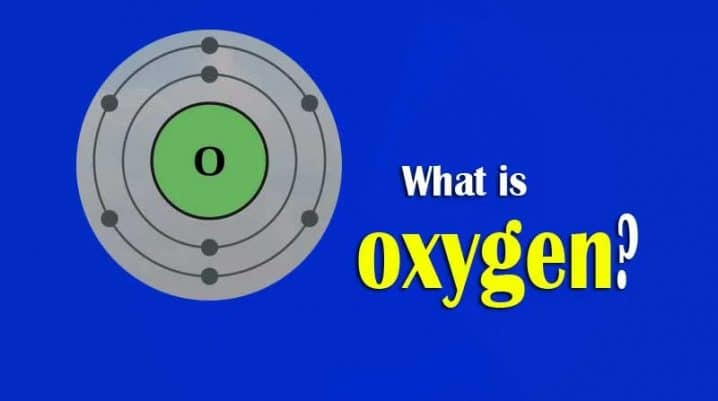# How To Find The Molar Mass Of a Molecule Of Oxygen (O2)?Oxygen is one of the most abundant elements in the universe and is only next to nitrogen in the atmosphere. Knowing the molar mass of oxygen is very important. So, for this, we need to know what molar mass is and how it is calculated while solving numericals in physical chemistry. Consequently, we will realize its importance. The atomic number of oxygen is 8. It has a molar mass of roughly 15.9994. The molar mass of individual elements helps in calculating compounds as well. For instance, nitrogen or N2 has a mass which is the total of the atomic masses of 2 individual nitrogen atoms- N. So, for NItrogen the atomic mass is 14.01 X 2 = 28.02 amu. Here 14 is the atomic mass of an individual Nitrogen atom.

The atomic mass of an element is the cumulative addition of all the atomic masses of the atoms it contains. What results from this is the molecular mass of the concerned molecule. Therefore, by that logic, the molar mass of a N2 molecule is 28.02 grams per mole. However, we can’t apply the term “molar mass” while considering non-molecular or ionic compounds. In such cases, we use the term “formula mass”. This is because ionic compounds cannot dissociate into individual molecules. However, the term molar mass holds if we are considering a mole of the concerned ionic compound. So, Calcium hydrogen carbonate (Ca(HCO3)2) will have a formula mass of 117.10 amu. Its molar mass will be 117.10 grams per mole.## How To Define Molar Mass?

The definition of the molar mass of a chemical substance is a little complex. It is the mass one mole of that element or compound possesses. However, in the question of moles, the definition does not explain much. A mole, therefore, is a unit that helps in measuring and stating the amount of matter a given substance contains.

Scientists use the concept of moles to calculate the number of elements that are present in a particular sample of chemicals. Atoms and molecules are the smallest units of elements and compounds respectively. Therefore, one mole of carbon contains the same number of elementary entities as the number of atoms. This is because it is an element. The number of atoms present is 6.02214076 X 10^23. Scientists call this Avogadro’s number after Avogadro’s name.

This Avogadro’s number, along with mole, are very foundational concepts in any calculations in physical chemistry. This is because there can be millions of chemical reactions. Each chemical reaction involves an array of atoms that interact with each other differently under different conditions. Thousands of rearrangements form. Therefore, representing such millions of atoms is quite impossible.

However, even though it is very difficult, scientists still need a unit of measurement to represent all these elementary entities. So, moles are used in chemical calculations. Avogadro’s number represents 1 mole. By using it, scientists can compare the weight with the number of atoms that the substance contains. The latter is the number of moles. One calculates it this way as keeping in mind the weight is much easier than the number of individual atoms in every chemical reaction.### Why is Avogadro’s Number So Important Even To Calculate The Molar Mass of Oxygen?

One has to use Avogadro’s number and the concept of moles when the calculations engage more than one kind of molecule. To understand the process and quantity of chemicals reacting, one needs Avogadro’s number. It also shows molecular interaction within a chemical reactant. Ensuing is an example that shows how moles make chemical representation and calculation easier.

There are two atoms of hydrogen and one atom of oxygen in every molecule of water. Therefore, one mole of water contains one mole of oxygen with two moles of hydrogen. We write this relationship as-

1 mole of H2O = 2 × 6.02214076 × 1023 of Hydrogen + 6.02214076 × 1023 of Oxygen.

So, to summarize, the molecular weight will be ideally equal to the mass that one mole of the given substance contains. For example, water has a molecular weight of 18.015 amu. Consequently, one mole of water weighs around 18.015 grams.

### How To Mathematically Calculate The Molar Mass Of Oxygen?

The molecular mass of an element is the total of the masses of the elements it contains. For calculating the molecular mass, one has to at first multiply the atomic mass of an element with the number of atoms present inside that molecule. Following this, one has to add all the masses of the elements that are present inside the molecule.

1 molecule of oxygen, O2, has two atoms.

Now, an oxygen atom has a mass of 16 amu.

A molecule of O2 has a mass of 2 × 16 = 32 amu.

Therefore, the Mass of one molecule of oxygen

⇒ 32/ Avogadro constant ( 6.02214076 × 1023)

⇒ 5.31 × 10-23g.